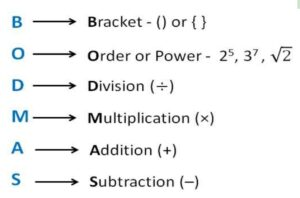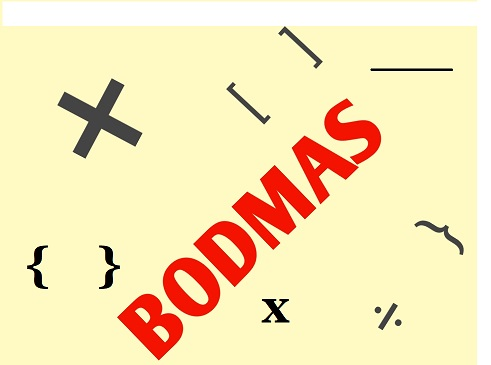December 8, 2023

# BODMAS Questions for class 5

BODMAS Questions for class 5

The abbreviation BODMAS rule is used to remember the procedures to be carried out in the right order while solving mathematical expressions. BODMAS stands for Brackets, Orders or Powers, Division, Multiplication, Addition, and Subtraction. Therefore, in BODMAS, the orders or exponents are given the second choice. We then carry out the arithmetic operations. A variant of BODMAS that is used in some regions is PEMDAS (Parentheses, Exponents, Multiplication, Division, Addition, and Subtraction).

The BODMAS rule states that if an expression involves brackets ((), {}, []), we must first solve or simplify the bracket before moving on to the left-to-right operations of division, multiplication, addition, and subtraction. A wrong answer will  be obtained if the problem is solved incorrectly.The following questions  on simplifications has different types of questions that can be practiced by the students to get more ideas to simplify the expressions.

Contents

# BODMAS QUESTIONS for class 5  with answers (Solved examples)

(i) 5 x 50 +57 -57 ÷ 57

Sol. 5 x 50 +57 -57 ÷ 57= 5 x 50 +57 -1                     (performing division first)

=250+57-1                             ( performing multiplication)

= 306

(ii) 4+4+4+4 ÷ 4

Sol. 4+4+4+4÷ 4  =4+4+4+1                                 (solving division)

= 13

(iii) 80+[20 x { 20-(10 ÷5) }]

Sol.  80+[20 x { 20-(10 ÷5) }] = 80+[20 x { 20-2 }]                    (Solving the small brackets)

=80+[20 x 18]                              (removing braces)

=  80+360                                      ( performing multiplication)

=440

(iv)  10 x 10 +[400 ÷ {100-(50-

)}]

Sol. 10 x 10 +[400 ÷ {100-(50-)}] = 10 x 10 +[400 ÷ {100-(50-30)}]                 (removing bar)

= 10 x 10 +[400 ÷ {100-20}]                          (removing small brackets)

= 10 x 10 +[400 ÷ 80]                                        ( removing braces)

= 10 x 10 +5                       (removing big brackets and performing division)

= 100+5                               (performing multiplication)

=105

(v) [{64-(12+13)}÷3]+15

Sol.  [{64-(12+13)}÷3]+15 = [{64-25}÷3]+15                               (removing small brackets)

=[39÷3]+15                                           (removing braces)

= 13+15                                                 (performing division)

=28

(vi) 7614 +832  x 48  ÷ 16- 8

Sol. 7614 +832  x 48  ÷ 16- 8 =7614 +832  x 3- 8         (performing division)

=7614 +2496- 8               ( performing multiplication)

= 10110-8

= 10102

(vii)  12- [20 ÷{8-2(9-5-2)}]

Sol.   12- [20 ÷{8-2(9-5-2)}]  =12- [20 ÷{8-2 x 2}]                    (solving expression in small brackets)

=12- [20 ÷{8-4}]

=12- [20 ÷4]                                   (removing braces)

= 12  -5                                              (removing big brackets)

=7

(viii) 25-[16-{5+18÷(4-

)}]

Sol. (viii) 25-[16-{5+18÷(4-)}]=25-[16-{5+18÷(4-2)}]                       (removing bar)

= 25-[16-{5+18÷2}]                            (removing small brackets)

= 25-[16-{5+9}]                                  (solving division inside curly braces)

=25-[16-14]

=25-2

=23

(ix) 15-[16-{12+21÷(9-2)}]

Sol. 15-[16-{12+21÷(9-2)}]=15-[16-{12+21÷7}]

=15-[16-{12+3}]

=15-[16-15]

=15-1

=14

(x)

Sol.

(xi)
Solution  :

= 118-9
=109

(xii) Evaluate: {15 × 32 ÷ 2 × 5} ÷ 75
Sol.  {15 × 32 ÷ 2 × 5} ÷ 75 = {15 × 16 × 5} ÷ 75
= {1200} ÷ 75
= 16

(xiii)

Sol:
Start by solving the innermost bar bracket
=

=1

‘BODMAS’  Simplification Questions for class 5  (UNSOLVED Examples)

Simplify

(i)  12+12 ÷ 3

(ii) 16 x 10÷ 2

(iii) 24-8 x 2

(iv) 32 ÷ 8+4 x 6-2

(v) 100-72 ÷ 8+4 x 3

(vi) 30+75  ÷ 15 x 4 -18

(vii) 8 x 6 +24 ÷ 6- 18

(viii) 56- 36 ÷ 4 x 2 +7

(ix) 56 ÷ 14 x 3 -10 ÷5 +1

(x) 198-42 x 15 ÷ 5 +6

(xi) 105 x 14 ÷ 7 +6 -1

(xii) 17+34 ÷ 17 x 5 -20

(xiii)  289+ 153 ÷ 17-8 x 19

(xiv) 7614+832 x 48 ÷16-8

(xv) 7823-128 ÷16 of 4 -3973

(xvi)

Ans. (i) 16   (ii) 80   (iii) 8    (iv) 26     (v) 103     (vi) 32      (vii) 34    (viii)  47    (ix) 11   (x) 78    (xi) 215     (xii) 7  (xiii) 146

(xiv) 10102    (xv) 3848     (xvi) 1

#### BODMAS QUIZ FOR CLASS 5

Click ‘Start Quiz’ to begin!

189
Created on ByBina singhBODMAS QUIZ CLASS 5

1 / 10

(10+43-5) ÷6 +

2 / 10

(19-8) x (10+4) +

3 / 10

2-20÷ 5 x 3

4 / 10

÷ (24-4)

5 / 10

1.   14+18 ÷2 x 18 -7

6 / 10

8 x 4 +9 -9+18

7 / 10

11 x 11 - 6 x 17 +4

8 / 10

5 x 50+57-57÷ 57

9 / 10

80+[20 x {20-(10÷5)}]

10 / 10

4+4+4+4÷ 4

The average score is 48%

0%

You might also be interested in:#### Bina singh

View all posts by Bina singh →
Last Minute Preparation Tips for SSC CGLTier II 2023 ssc chsl 2023 tier 1 cut off NIRF Rankings 2023 : Top 10 Engineering colleges in India CBSE Compartment Exam 2023 Application Form SSC CGL 2023 Notification: Important Dates